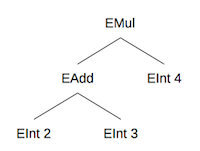# Module 04 Project

## Introduction

In this PA you will implement some analysis routines over a data structure called an expression tree. You will implement similar projects in Ruby, Haskell, and Prolog, and the goal is to explore the differences between the three languages.

In Ruby, you will extend existing Ruby classes to practice dynamic object-oriented programming. In Haskell, you will write pattern-matching routines to practice functional programming. In Prolog, you will write rules to practice logical programming.

Project starter files: `p2.zip`

## Requirements

You may ignore `lexer.rb` and `parser.rb`. They were auto-generated from grammar specification files using utilities called Rexical and Racc, and they provide the backend code for parsing simple mathematical expressions. Here is a context-free grammar describing the language that this parser can recognize:

``````    E -> <int>
| E + E
| E - E
| E * E
| ( E )
``````

You will want to take a look at the definitions in `defs.rb`, which defines the following class hierarchy:

• Expr
• EInt
• EBinOp
• ESub
• EMul

These classes represent nodes in the expression parse tree. `Expr` and `EBinOp` are parent classes that for the purposes of this project should be considered abstract. `EInt` represents an integer leaf node, while the other three represent interior arithmetic operation nodes. For instance, the expression `(2+3)*4` will be parsed into the following tree:Your task in this PA will be to extend the provided expression tree node classes to analyze expression trees. Because class definitions in Ruby are dynamic, you can add new members to a class definition at any point by providing another class definition with the new members.

For example, when placed in `p2.rb`, the following code will add a new `min_int` function to all concrete expression node classes (recall that any subclasses that do not override a method will inherit the parent class’s implementation):

``````class EBinOp
def min_int
[left.min_int, right.min_int].min
end
end

class EInt
def min_int
@val
end
end
``````

Below are descriptions of the methods you need to add. You can also look at the test cases to see what they are supposed to do. You should think about what the definition of each function means for each expression tree node type, and you certainly do not need to implement every method in every class.

• `eval` - Calculates the result of evaluating the mathematical expression. All operations should be done using integer arithmetic.
• `count_ops` - Counts the total number of arithmetic operations in an expression. All three operations (addition, subtraction, and multiplication) count for this process.
• `height` - Calculates the height of the expression tree, where the height of a single-node tree is defined as being 1.
• `postfix` - Returns a flattened postfix string representation. For example, `(2+3)*4` in postfix notation would be `"2 3 + 4 *"`. You should use the `to_s` method to convert integers to strings.
• `uniq_ints` - Extract a sorted list of all unique integers in an expression. Each integer should appear in the resulting list only once.

Some test routines are included in `test.rb`. However, your submission will be graded based on additional tests not provided to you, so you should write your own test cases as well. You can also test your routines via the provided REPL (read/eval/print loop) by running `main.rb`.

For this assignment, you may NOT use any classes or methods that are not in the Ruby Core (i.e., you shouldn’t `require` anything). If you are unsure about a particular class or method, you can consult the documentation. Also, we will test your submission using Ruby 2.6 so you shouldn’t depend on any features added after that version.

## Submission

Your program must contain implementations as described above. You must name your Ruby script file `p2.rb`. You must put your name in a comment at the top of the file. As usual, decompose your methods to make them more readable, use good names, indent properly (indentation is conventionally two spaces in Ruby), and so on. You will partly be graded on the readability of your code but mostly on whether it works.

Submit ONLY `p2.rb` to the appropriate assignment on Canvas by the deadline.